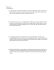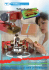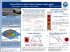# Static: F ` Âµ F Kinetic F ` Âµ F Metal on metal 0.15

## Transcription

Static: F ` Âµ F Kinetic F ` Âµ F Metal on metal 0.15
```Friction
Normal and Tangential Axes
•
normal axis is perpendicular to surface
•
tangential axis is parallel to surface
tangent line
to surface
Y
X
Laws of Dry Friction:
Static:
Kinetic
Fstatic ' µstatic Fnormal
Fkinetic ' µkinetic Fnormal
Coefficients of Static Dry Friction
Metal on metal
Metal on wood
Metal on stone
Wood on wood/leather
Stone on stone
Earth on earth
Rubber on concrete
Nylon on nylon
Bone on bone (cartilage)
Steel on Teflon
Metal of ice
23
From: Robertson, Chapter 4
0.15 - 0.60
0.20 - 0.60
0.30 - 0.70
0.25 - 0.50
0.40 - 0.70
0.20 - 1.00
0.60 - 0.90
0.15 - 0.25
0.10 - 0.20
0.04 - 0.05
0.02 - 0.05
Forces of Friction:
Fapplied
W = weight
Ffriction
Fnormal
Example:
Free-body diagram
Space diagram
Fapplied
Fapplied
θ = 20E
θ = 20E
W
Ffriction
n
+
24
From: Robertson, Chapter 4
Fnormal
t
Relationship between Applied Force and Friction:
•
•
•
•
as applied force increases friction increases until a
maximum is reached and slipping occurs
maximum is called Fstatic
after body starts to move frictional force drops to a
new level called Fkinetic
any further increase in the applied force is resisted by
Fkinetic
Ffriction
point of
impending
motion
Fstatic
Fkinetic
Fapplied
25
From: Robertson, Chapter 4
Empirical Method for Calculating Coefficient of Static
Friction:
•
•
•
•
•
•
•
•
cover a load and incline with two surfaces to be tested
place load on an incline that can be raised at one end
make sure incline and load are flat and clean
increase incline until load just starts to slip
measure angle of incline, θ
repeat and obtain average angle
coefficient of static friction = µstatic = tan θ
proof follows
W = weight
Ffriction
θ
θ
Fnormal
At instant of impending motion:
•
•
26
µstatic= tan θ
coefficient of kinetic friction is more difficult to obtain
tan of angle that keeps load moving at constant velocity
From: Robertson, Chapter 4
Angles of Friction
•
•
angle of an incline at the point of impending motion
tangent (tan) of this angle is the same as the coefficient of
static friction
W = weight
Ffriction
θ
θ
Fnormal
At instant of impending motion:
µS = tan θ
Proof:
∑ Fn = 0: Fnormal − W cos θ = 0
Fnormal = W cosθ
∑ Ft = 0: Fstatic − W sinθ = 0
Fstatic = W sinθ
Fstatic W sinθ
µ static =
=
= tan θ
Fnormal W cosθ
•
•
27
to compute the coefficient of kinetic friction, lower the
incline slowly until the mass just stops–this is the angle of
kinetic friction
as above the tan of this angle is the coefficient of kinetic
friction
From: Robertson, Chapter 4
Frictional States
(a) no friction
(b) no motion (Fx < Fstatic)
Fapplied
Fy
Fapplied
Fx
W = weight
W = weight
Ffriction
F normal
Fnormal
(c) motion impending (Fx = Fstatic) (d) motion (Fx > Fstatic)
Fapplied
Fy
Fx
Fx
W = weight
F static
W = weight
Fkinetic
F normal
Fnormal
28
Fapplied
Fy
From: Robertson, Chapter 4
Measuring Friction using Force Platforms
•
•
•
•
•
•
line load and force platform with surfaces to be tested
pull load across clean level force platform
record maximum horizontal force (Fx) at point load
starts to move
µstatic = horizontal force / vertical force
record horizontal force when load is moving
µkinetic = horizontal force / vertical force
Fapplied
Fnormal = Fy
W = weight
material
tested
F friction = Fx
force platform
29
From: Robertson, Chapter 4
```

### RH572020 0315 - The Raybestos Aftermarket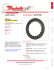### Exam 2 Solutions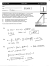### PROFILES PROJECT AMBROSI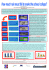### HabaCHAIN TS Material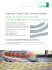### Simple Friction Experiments - Veletrh nápadů učitelů fyziky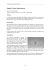### Symmetry-breaking due to Coulomb friction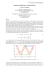### Physics 111 SI Worksheet 4 1. A 20 kg box sits on a horizontal